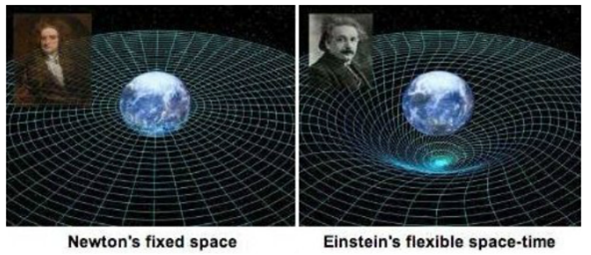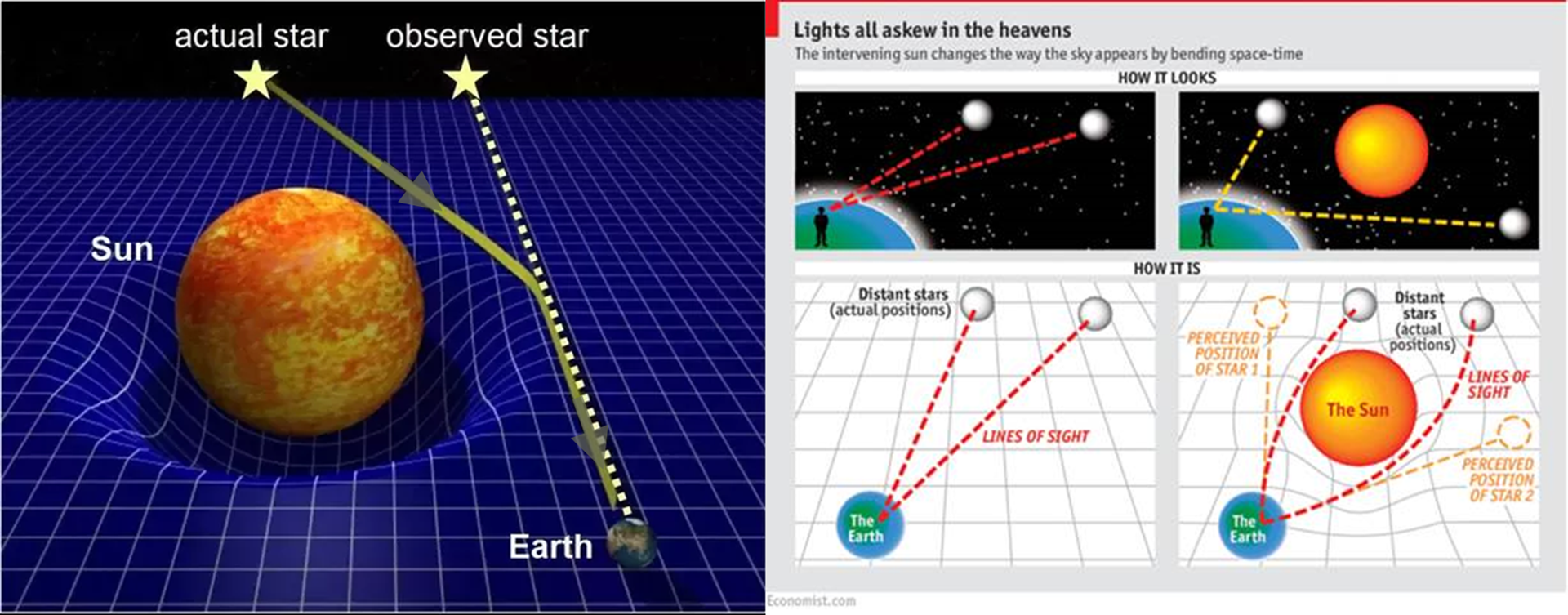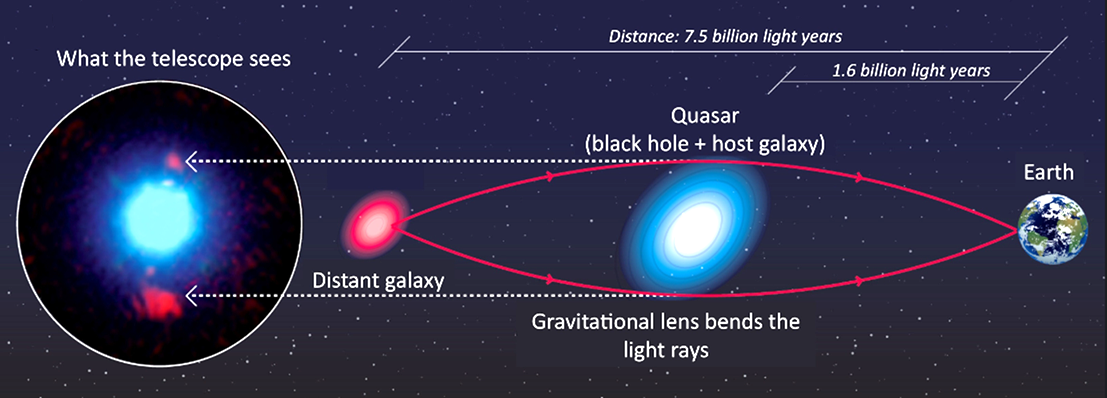Is light deflected in a universe of 100 billion galaxies of 1000 stars?
 Last time we talked about the diffraction and interference of light on 22 August, and in this report, let's talk about gravitational deflection of light in space. 1. What is gravity? First, let’s talk about Newtonian gravity and Einstein’s gravity. Newton defines that gravity as the attractive attraction between two objects with mass. But Einstein explains gravity as a distortion of space caused by the matter with mass. In other words, Newton states that there is only the attractive force between two objects without the spatial distortion around the objects. But Einstein states that a massive object makes spatial distortion which is defined as gravity.Figure 1. Newton and Einstein's Definition of Gravity
 2. Light deflection and gravitational lens effect Einstein predicted that the light path is changed by spatial distortion caused by matter such as the sun based on general relativity, as shown in figure 2. And, Arthur Eddington proved experimentally Einstein's gravity theory was correct by confirming that the observed position were different from the actual position because the light passing near the sun was deflected.Figure 2. Light deflection by the sun
 In this way, light deflection by the gravity of a massive matter is called Gravitational lens effect and it can be seen during astronomical observation. For example, as shown in figure 3, light emitted from distant galaxy are bent by massive quasar's gravity and observer on earth sees it as if there is a red ring around the quasar.Figure 3. Gravitational lens effect
 SungSoo Kim Ph.D Physicist * Next week’s topic: Effects of Artificial Lighting on BirdsYou can find "Interesting Story about Light Science” series on the link below.
 Seoul Semiconductor / ohc@seoulsemicon.com 97-11, Sandan-ro 163beon-gil, Danwon-gu, Ansan-si, Gyeonggi-do, Republic of Korea Unsubscribe